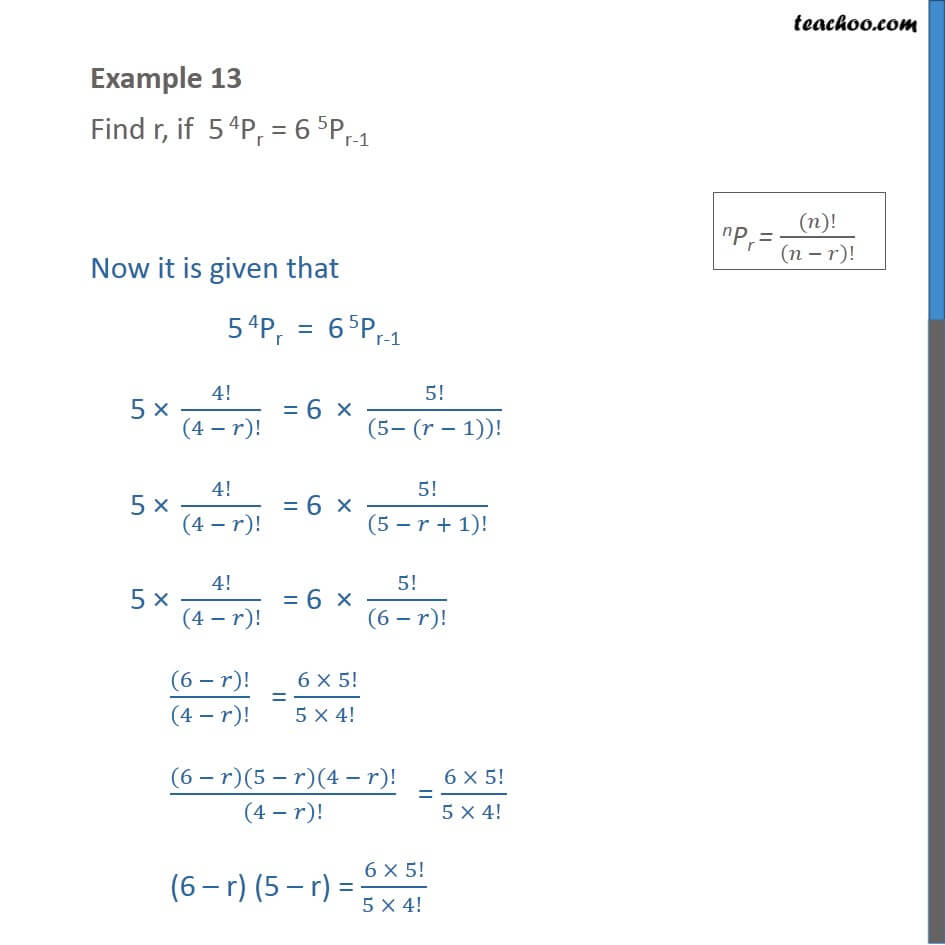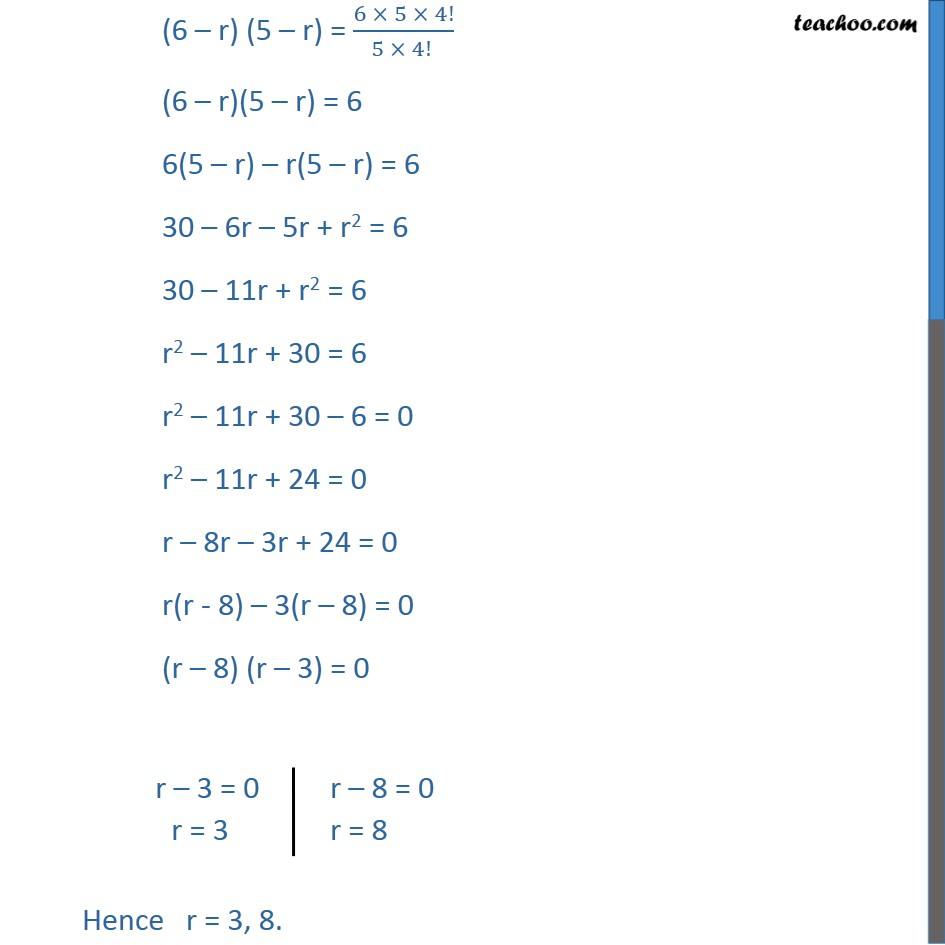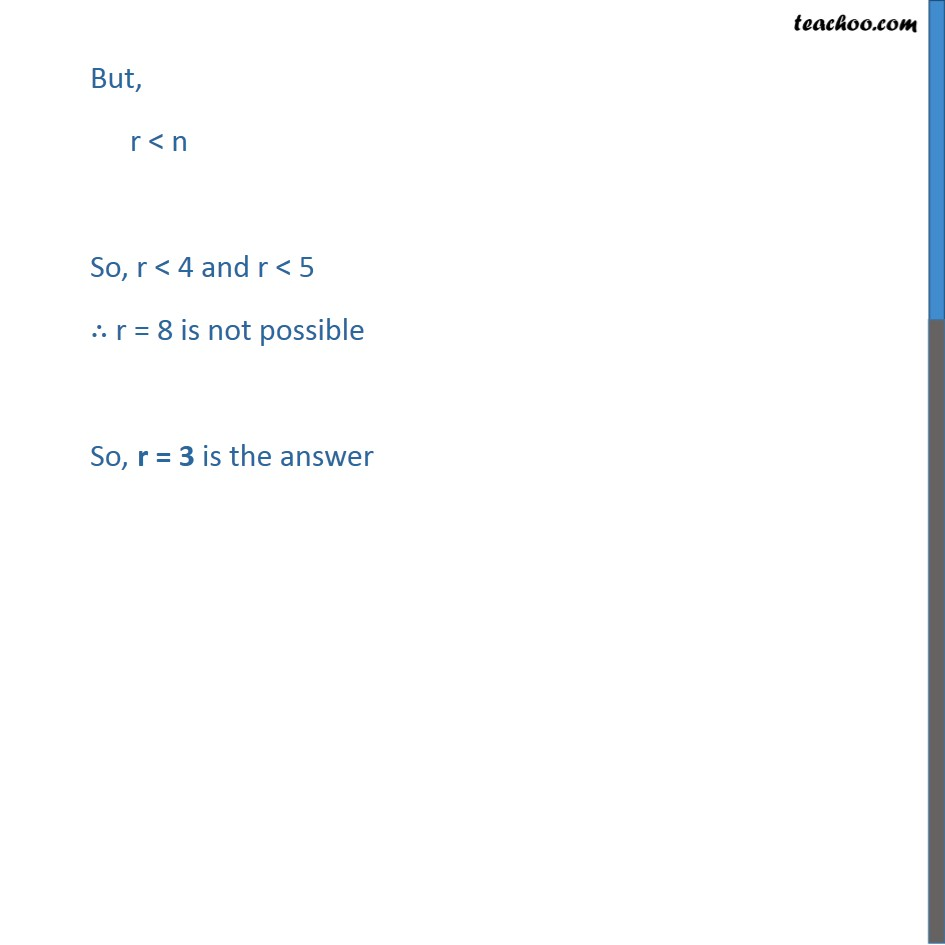1. Class 11
2. Important Question for exams Class 11
3. Chapter 7 Class 11 Permutations and Combinations

Transcript

Example 13 Find r, if 5 4Pr = 6 4Pr-1 Now it is given that 5 4Pr = 6 5Pr-1 5 × 4!/(4 − 𝑟)! = 6 × 5!/(5− (𝑟 − 1))! 5 × 4!/(4 − 𝑟)! = 6 × 5!/(5 − 𝑟 + 1)! 5 × 4!/(4 − 𝑟)! = 6 × 5!/(6 − 𝑟)! (6 − 𝑟)!/(4 − 𝑟)! = (6 × 5!)/(5 × 4! ) ((6 − 𝑟)(5 − 𝑟)(4 − 𝑟)!)/(4 − 𝑟)! = (6 × 5!)/(5 × 4! ) (6 – r) (5 – r) = (6 × 5!)/(5 × 4! ) (6 – r) (5 – r) = (6 × 5 × 4!)/(5 × 4! ) (6 – r)(5 – r) = 6 6(5 – r) – r(5 – r) = 6 30 – 6r – 5r + r2 = 6 30 – 11r + r2 = 6 r2 – 11r + 30 = 6 r2 – 11r + 30 – 6 = 0 r2 – 11r + 24 = 0 r – 8r – 3r + 24 = 0 r(r - 8) – 3(r – 8) = 0 (r – 8) (r – 3) = 0 Hence r = 3, 8. But, r < n So, r < 4 and r < 5 ∴ r = 8 is not possible So, r = 3 is the answer

Chapter 7 Class 11 Permutations and Combinations

Class 11
Important Question for exams Class 11

About the AuthorDavneet Singh
Davneet Singh is a graduate from Indian Institute of Technology, Kanpur. He has been teaching from the past 9 years. He provides courses for Maths and Science at Teachoo.